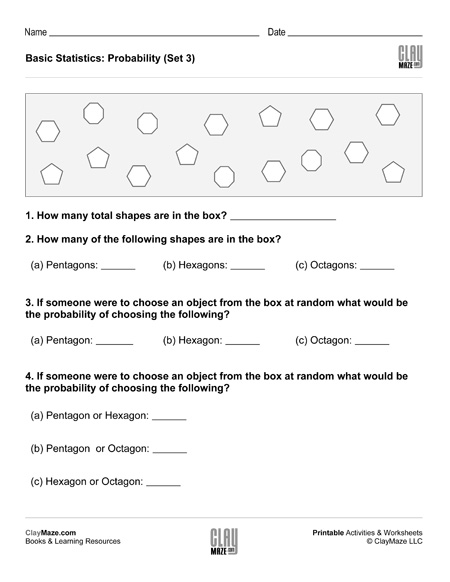Basic Statistics: Probability I (Set 3)

This is a basic probability worksheet. The questions are based on the number of shapes in the box and the student is asked to find the probabilities of randomly choosing the different shapes.